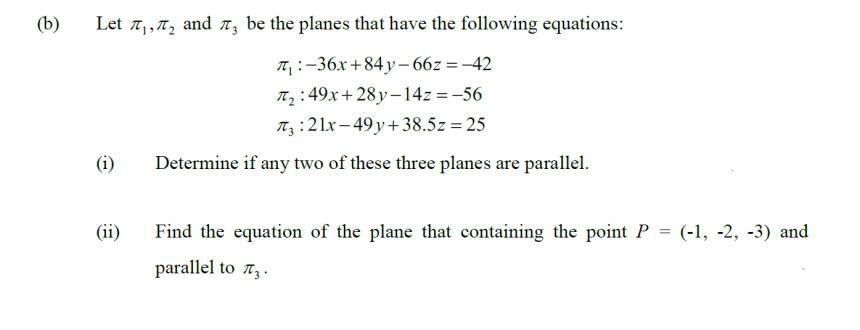Question:

# (b) = Let , 12 and 13 be the planes that have the following equations: T.:-36x +84 y - 66z = -42 12:49x + 28y-14z = -56 Itz: 2 l(b) = Let , 12 and 13 be the planes that have the following equations: T.:-36x +84 y - 66z = -42 12:49x + 28y-14z = -56 Itz: 2 lx-49 y + 38.5z = 25 (i) Determine if any two of these three planes are parallel. (ii) Find the equation of the plane that containing the point P = (-1, -2, -3) an parallel to tz.